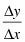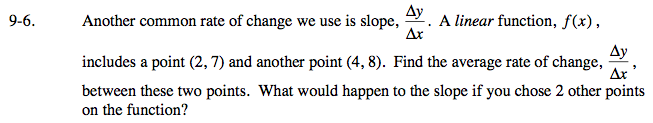### Home > AC > Chapter 16 > Lesson 16.9.1.1 > Problem9-6

9-6.

Another common rate of change we use is slope,. A linear function, f(x), includes a point (2, 7) and another point (4, 8). Find the average rate of change,, between these two points. What would happen to the slope if you chose 2 other points on the function? Homework Help ✎$\frac{\Delta\textit{x}}{\Delta\textit{y}}=\frac{y_{2} - y_{1}}{x_{2} - x_{1}}$